# 灰条

Graystripe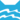河族 (Q630)[注 1]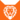狮族 (Q646)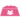宠物猫 (Q647)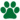独行猫 (Q648)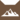急水部落 (Q638)[临时]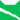雷族 武士河族 武士雷族 武士雷族 副族长
（继任自白风 (Q715)雷族 族长[注 2]雷族 武士雷族 长老雷族 族长[注 3]
（继任自狮焰 (Q3093)雷族 长老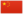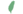灰崽非官 Graykit  小灰非官
 灰爪 Graypaw  灰掌
 灰条 Graystripe  灰紋

Q714：雷族公猫，自《呼唤野性》登场的角色

## 细节

### 外貌

• 他是一只身形庞大、:67皮毛粗长、:13黄色眼睛:14的纯:猫物表暗灰色长毛公猫，:40背上有一条颜色更深的条纹，左耳撕裂，:143颈毛厚实。:123

### 趣闻

• 他具有天族血统（来自蝰蛇牙）。
• 儿子暴毛的外貌和他极为相似。:239-240
• 儿子黄蜂条的外貌和他极为相似，但有着比他更多的条纹。[注 5]:158
• 他是唯一一只三度担任副族长的猫，:297虽然后面两次只是在黑莓掌前往急水部落和寻找日神时代理职务。:146:3章
• 他是两度担任族长的猫之一，分别在火星离开雷族重建天族时代理职务，:10章以及接替狮焰成为代理族长。:91
• 他是在退休为长老后成为族长的猫之一。:91
• 外曾孙[注 6]小灰的名字可能是为了纪念他。
• 小灰和同窝手足的名字前缀正好和父亲茎叶:4章表姐[注 7]鬃霜:18章及太外公灰条:22章相同，而这三只猫都因蜡毛造成的混乱而牺牲。

## 登场书目

• 呼唤野性 (Q57)[主要角色][首次登场🐱]
• 寒冰烈火 (Q59)[主要角色]
• 疑云重重 (Q60)[配角]
• 风起云涌 (Q65)[配角]
• 险路惊魂 (Q66)[配角]
• 力挽狂澜 (Q67)[配角]
• 午夜追踪 (Q139)[配角]
• 新月危机 (Q140)[配角]
• 重现家园 (Q141)[配角]
• 星光指路 (Q142)[对话提及]
• 黄昏战争 (Q143)[对话提及]
• 日落和平 (Q144)[对话提及]
• 预视力量 (Q152)[配角]
• 暗河汹涌 (Q153)[配角]
• 驱逐之战 (Q154)[配角]
• 天蚀遮月 (Q155)[配角]
• 暗夜长影 (Q156)[配角]
• 拂晓之光 (Q157)[配角]
• 第四学徒 (Q158)[配角]
• 战声渐近 (Q159)[配角]
• 暗夜密语 (Q160)[配角]
• 月光印记 (Q161)[配角]
• 武士归来 (Q162)[配角]
• 群星之战 (Q163)[配角]
• 学徒探索 (Q170)[配角]
• 雷影交加 (Q171)[配角]
• 天空破碎 (Q172)[配角]
• 极夜无光 (Q173)[配角]
• 烈焰焚河 (Q174)[配角]
• 风暴来袭 (Q175)[配角]
• 迷失群星 (Q3563)[配角]
• 静默冰融 (Q3579)[配角]
• 叠影重障 (Q3624)[配角]
• 暗由心生 (Q4183)[配角]
• 无星之地 (Q4385)[配角]
• 迷雾明光 (Q4621)[配角][死亡时刻✝]
• 火星的探索 (Q176)[配角]
• 蓝星的预言 (Q177)[配角]
• 钩星的承诺 (Q179)[配角]
• 黑莓星的风暴 (Q182)[配角]
• 虎心的阴影 (Q185)[猫物闲角]
• 鸦羽的拷问 (Q186)[配角]
• 松鼠飞的希冀 (Q3561)[配角]
• 灰条的誓言 (Q4057)[主人公][主要角色][视角人物]
• 豹星的荣耀 (Q4426)[配角]
• 冬青叶的故事 (Q187)[猫物闲角]
• 雾星的征兆 (Q188)[配角]
• 虎掌的愤怒 (Q190)[配角]
• 叶池的祈愿 (Q191)[对话提及]
• 鸽翅的沉默 (Q192)[配角]
• 乌爪的告别 (Q195)[对话提及]
• 红尾的恩债 (Q3565)[配角]
• 褐皮的族群 (Q3566)[猫物闲角]
• 蛾翅的秘密 (Q3628)[猫物闲角]
• 黛西的至亲 (Q3629)[对话提及]
• 斑毛的叛变 (Q4337)[配角]
• 黑脚的清算 (Q4336)[对话提及]
• 河族阴云 (Q4311)[配角]
• 变革之风 (Q4411)[对话提及]
• 逐出影族 (Q4769)[配角]
• 武士失踪 (Q207)[主人公][主要角色][视角人物]
• 武士避难 (Q208)[主人公][主要角色][视角人物]
• 武士回归 (Q209)[主人公][主要角色][视角人物]
• 和平破碎 (Q213)[仅于回忆]
• 族群救星 (Q214)[配角]
• 武士之心 (Q215)[配角]
• 族群的猫 (Q200)[配角]
• 族群的守则 (Q201)[配角]
• 族群的战争 (Q202)[配角]
• 终极指南 (Q204)[配角]
• ## 周边

更多资料：灰条/周边
【系列1】灰条
• 系列1——火星、灰条、松鸦羽
• 灰条是自己图像小说三部曲的主演，是《猫武士》系列最受欢迎的角色之一，现在欢庆他的娃娃实体化！灰条的大绒毛娃娃包含了这只雷族猫暗灰色皮毛上那与众不同的条纹，以及他脚掌上的特殊族群标志。
• 约29.99美金。

【系列1】松鸦羽和灰条
• 系列1——火星和叶星、松鸦羽和灰条、虎星褐皮
• 灰条和松鸦羽是雷族最重要的两只猫之一——灰条的仁慈、忠诚，以及对他族猫的奉献；松鸦羽的治疗技巧、他的预言幻象，还有当然，他那独一无二的看护礼仪。
• 长约7厘米。
• 一组约12.99美金；整套三组约38.97美金。

## 脚注

1. 因放逐而离开
2. 原因：天族重建 (Q3676) - 在《火星的探索》中，火星和沙风重建现代天族的过程
3. 原因：黑莓星被附身 (Q4384) - 在《破灭守则》中，黑莓星被附身假冒的事件
4. 他的武士美德之一是力量strength），在正体中文版中错译为“胆识”。
5. 尽管书中如此描述黄蜂条，但实际上灰条只有一条脊背上的条纹。
6. 小灰是他的外孙茎叶的儿子。
7. 鬃霜是他的舅舅香薇歌的女儿。

## 参考文献

这篇文章基于CC BY-SA 3.0许可使用了猫武士维基（英语）Graystripe一文中的部分内容。
1. 《迷雾明光》第二十二章 (Q4792)
2. 《呼唤野性》第一章 (Q741)
3. 某时间点 — 《呼唤野性》第一章 (Q741)
4. Kate (2016-03-06). 站内截图. BlogClan . 原页面 归档于 2018-04-04.
5. Official Website (2019-01-07). Family Tree . WarriorCats.com .
6. 一星的告解 (Q4826)
7. 《武士失踪》第三章 (Q2257) — 某时间点
8. 寒冰烈火
9. 呼唤野性
10. 预视力量
11. 迷雾明光
12. Su Susann (2016-09-30). 站内截图. Facebook . 原页面 归档于 2017-10-31. “As I said, Adderfang is a descandant of Spottedpelt and Pinestar is, trough the blood of his father, related to Gorseclaw.”
13. 日落和平
14. 天蚀遮月
15. 力挽狂澜
16. 驱逐之战
17. 拂晓之光
18. 火星的探索
19. 无星之地
20. 暗由心生